Test: Random Process

# Test: Random Process

Test Description

## 20 Questions MCQ Test GATE ECE (Electronics) 2023 Mock Test Series | Test: Random Process

Test: Random Process for Electronics and Communication Engineering (ECE) 2023 is part of GATE ECE (Electronics) 2023 Mock Test Series preparation. The Test: Random Process questions and answers have been prepared according to the Electronics and Communication Engineering (ECE) exam syllabus.The Test: Random Process MCQs are made for Electronics and Communication Engineering (ECE) 2023 Exam. Find important definitions, questions, notes, meanings, examples, exercises, MCQs and online tests for Test: Random Process below.
Solutions of Test: Random Process questions in English are available as part of our GATE ECE (Electronics) 2023 Mock Test Series for Electronics and Communication Engineering (ECE) & Test: Random Process solutions in Hindi for GATE ECE (Electronics) 2023 Mock Test Series course. Download more important topics, notes, lectures and mock test series for Electronics and Communication Engineering (ECE) Exam by signing up for free. Attempt Test: Random Process | 20 questions in 60 minutes | Mock test for Electronics and Communication Engineering (ECE) preparation | Free important questions MCQ to study GATE ECE (Electronics) 2023 Mock Test Series for Electronics and Communication Engineering (ECE) Exam | Download free PDF with solutions
 1 Crore+ students have signed up on EduRev. Have you?
Test: Random Process - Question 1

### Consider a low-pass random process with a white-noise power spectral density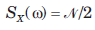as shown in fig. ​ ​ ​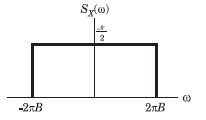Que: The auto correlation function Rx(τ) is

Detailed Solution for Test: Random Process - Question 1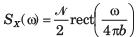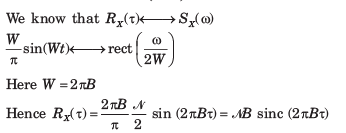Test: Random Process - Question 2

### Consider a low-pass random process with a white-noise power spectral densityas shown in fig.Que: The power PX is

Detailed Solution for Test: Random Process - Question 2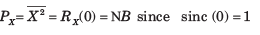Test: Random Process - Question 3

### If X(t) is a stationary process having a mean value E[X(t)] = 3 and autocorrelation function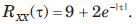The variance of random variable Y =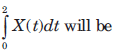Detailed Solution for Test: Random Process - Question 3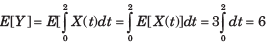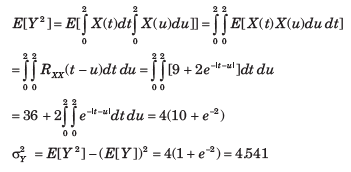Test: Random Process - Question 4

A random process is defined by X(t) = Acos(πt) where A is a gaussian random variable with zero mean and variance σπ2. The density function of X(0)

Detailed Solution for Test: Random Process - Question 4Test: Random Process - Question 5

The two-level semi-random binary process is defined by X(t) = A or -A

where (n-1)T < t < nt and the levels A and -A occur with equal probability. T is a positive constant and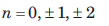Que: The mean value E[X(t)] is

Detailed Solution for Test: Random Process - Question 5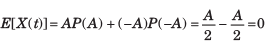Test: Random Process - Question 6

The two-level semi-random binary process is defined by X(t) = A or -A

where (n-1)T < t < nt and the levels A and -A occur with equal probability. T is a positive constant andQue: The auto correlation Rxx = (t1 = 0.5T, t2 = 0.7 T) will be

Detailed Solution for Test: Random Process - Question 6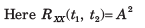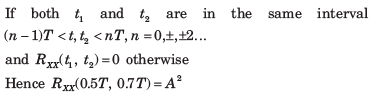Test: Random Process - Question 7

A random process consists of three samples function X(t, s1 ) = 2, X(t, s2 ) = 2cos t1  and X(t, s3 ) = 3sint  - each occurring with equal probability. The process is

Detailed Solution for Test: Random Process - Question 7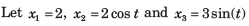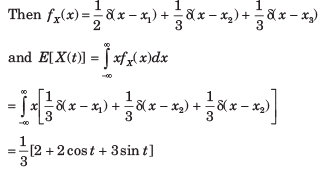The mean value is time dependent so X (t) is not stationary in any sense.

Test: Random Process - Question 8

The auto correlation function of a stationary ergodic random process is shown in fig.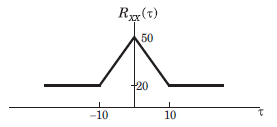Que: The mean value E[X(t)] is

Detailed Solution for Test: Random Process - Question 8

We know that for ergodic with no periodic component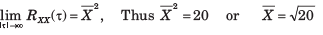Test: Random Process - Question 9

The auto correlation function of a stationary ergodic random process is shown in fig.Que: The E[X2(t)] is

Detailed Solution for Test: Random Process - Question 9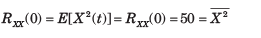Test: Random Process - Question 10

The auto correlation function of a stationary ergodic random process is shown in fig.Que: The variance σx is

Detailed Solution for Test: Random Process - Question 10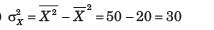Test: Random Process - Question 11

A stationary zero mean random process X(t) is ergodic has average power of 24 W and has no periodic component. The valid auto correlation function is

Detailed Solution for Test: Random Process - Question 11

For (A) : It has a periodic component.
For (B) ; It is not even in τ, total power is also incorrect.
For (C) It depends on t not even in τ and average power is ∞

Test: Random Process - Question 12

A stationary random process X(t) is applied to the input of a system for which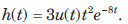If E[X(t)] = 2, the mean value of the system's response Y(t) is

Detailed Solution for Test: Random Process - Question 12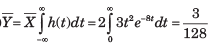Test: Random Process - Question 13

A random process X(t) is applied to a network with impulse response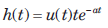where a > 0 is a constant. The cross correlation of X(t) with the output Y(t) is known to have the same form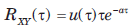Que: The auto correlation of Y(t) is

Detailed Solution for Test: Random Process - Question 13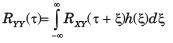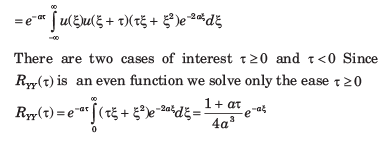Test: Random Process - Question 14

A random process X(t) is applied to a network with impulse responsewhere a > 0 is a constant. The cross correlation of X(t) with the output Y(t) is known to have the same formQue: The average power in Y(t) is

Detailed Solution for Test: Random Process - Question 14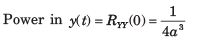Test: Random Process - Question 15

A random noise X(t) having a power spectrum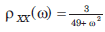is applied to a differentiator that has a transfer function H(ω) =  j(ω). The output is applied to a network for which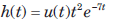Que : The average power in X(t) is

Detailed Solution for Test: Random Process - Question 15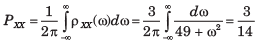Test: Random Process - Question 16

A random noise X(t) having a power spectrumis applied to a differentiator that has a transfer function H(ω) =  j(ω). The output is applied to a network for whichQue : The power spectrum of Y(t) is

Detailed Solution for Test: Random Process - Question 16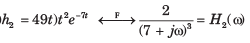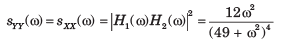Test: Random Process - Question 17

White noise with power density N0 /2 is applied to a low pass network for which |H(0)| = 2. It has a noise bandwidth of 2 MHz. If the average output noise power is 0.1 W in a 1 - Ω( resistor, the value of Nis

Detailed Solution for Test: Random Process - Question 17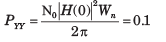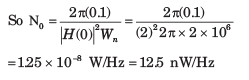Test: Random Process - Question 18

An ideal filter with a mid-band power gain of 8 and bandwidth of 4 rad/s has noise X(t) at its input with power spectrum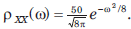The noise power at the network's output is (F(2) = 0.9773)

Detailed Solution for Test: Random Process - Question 18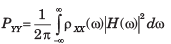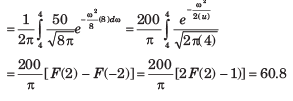Test: Random Process - Question 19

White noise with power density N0 /2 = 6 μW/Hz is applied to an ideal filter of gain 1 and bandwidth W rad/s. If the output's average noise power is 15 watts, the bandwidth W is

Detailed Solution for Test: Random Process - Question 19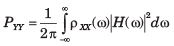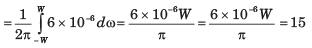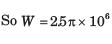Test: Random Process - Question 20

A system have the transfer function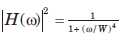where W is a real positive constant. The noise bandwidth of the system is

Detailed Solution for Test: Random Process - Question 20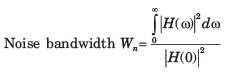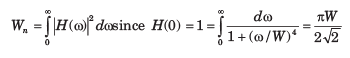## GATE ECE (Electronics) 2023 Mock Test Series

20 docs|261 tests
 Use Code STAYHOME200 and get INR 200 additional OFF Use Coupon Code
Information about Test: Random Process Page
In this test you can find the Exam questions for Test: Random Process solved & explained in the simplest way possible. Besides giving Questions and answers for Test: Random Process , EduRev gives you an ample number of Online tests for practice

## GATE ECE (Electronics) 2023 Mock Test Series

20 docs|261 tests